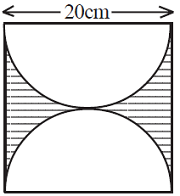# Unified Council - UIMO PDF Sample Papers for Class 10

Class 10 sample paper & practice questions for Unified International Mathematics Olympiad (UIMO) level 1 are given below. Syllabus for level 1 is also mentioned for these exams. You can refer these sample paper & quiz for preparing for the UIMO exam.#### Resources:

##### Sample Questions from Olympiad Success:
 Q.1 Q.2 Q.3 Q.4 Q.5 Q.6 Q.7 Q.8 Q.9 Q.10
 Q.1 A batsman played three matches in a tournament. The ratio of the score of 1st to 2nd match was 8: 9 and that of the score of 2nd to 3rd match was 3: 2. The difference between the 1st and the 3rd match was 16 runs. What was the batsman's average score in all three matches? a) 40 b) 58 c) 45 1/5 d) 61 1/3
 Q.2 A tangent to a circle (centre O) drawn from an exterior point T to the circle and touches the circle at a point P on the circumference. Which of the following statement is TRUE? a) ∠TPO > 90° b) ∠TPO < 180° c) ∠TPO < 90° d) ∠TPO = 90°
 Q.3 The median of the triangle ABC meets at point G, which of these is not true? a) Area of triangle AGC = Area of triangle BGC b) Area of triangle AGC = Area of triangle AGB c) Area of triangle AGC = (1/3) Area of triangle ABC d) None of these
 Q.4 A paper is in the form of a square of side 20 cm. Semicircle are down inside the square paper on two sides as diameter. The semi-circular portions are cut off. Find the remaining paper.a) 87 cm² b) 86.3 cm² c) 86 cm² d) 85.7 cm²
 Q.5 In a certain language, if "chi to le" means "We are citizen" and "chi to nu" means "We are nice", then what word represents the word "nice"? a) NU b) EE c) TU d) TO
 Q.6 At Bilal’s Discount Hardware everything is sold for 30% less than the market price. If Bilal’s buys tool bits for Rs. 96, what price should he marked them if he wants to make a 20% profit on his cost? a) 117 b) 127 c) 146 d) 164
 Q.7 In the probability of getting a number greater than or equal to 3 in a throw of a biased dice is 5/6, then how many of the six numbers in the biased dice are definitely less than 3? a) 2 b) 3 c) 1 d) Cannot be determined
 Q.8 Which of these is false? a) The diagonal of the parallelogram divide the parallelogram into four equal parts b) Area of parallelogram is base multiplied by the height c) If EFGH are the respective midpoints of the sides of the parallelogram ABCD, Area of EFGH is half the area of the parallelogram d) None of these
 Q.9 A dealer bought 80 cricket bats for ₹ 50 each. He sells 20 of them at a gain of 5%. What must be the gain percentage of the remaining bats, so as to get 10% gain on the whole? a) 3 2/11 % b) 12 1/2 % c) 11 2/3 % d) 4 2/3 %
 Q.10 If 4th and 8th terms of an A.P. are 11 and 23 respectively, find a and d. a) a = 2, d = 3 b) a = 2, d = 2 c) a = 3, d = 3 d) a = 1, d = 3Sample PDF of Unified Council - Unified International Mathematics Olympiad (UIMO) PDF Sample Papers for Class 10:

 Q.1 )d Q.2 )d Q.3 )d Q.4 )d Q.5 )a Q.6 )d Q.7 )b Q.8 )d Q.9 )c Q.10 )a

Q.1 : d | Q.2 : d | Q.3 : d | Q.4 : d | Q.5 : a | Q.6 : d | Q.7 : b | Q.8 : d | Q.9 : c | Q.10 : a